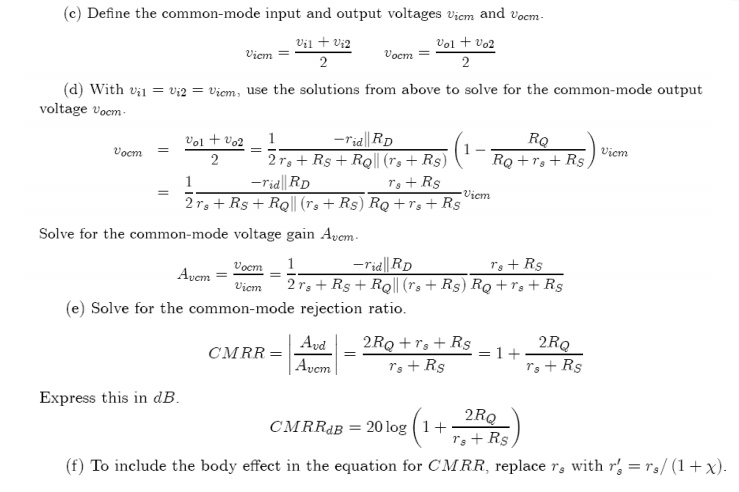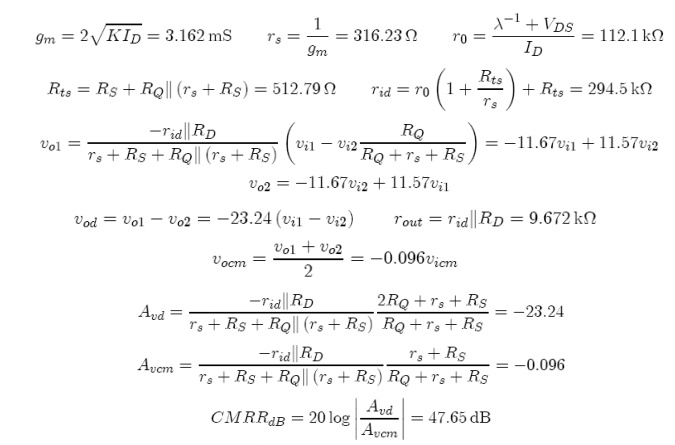Home | | Electronic Circuits I | MOSFET Differential amplifier

# MOSFET Differential amplifier

DC Solution, Small Signal or AC Solutions, Common-Mode Rejection Ratio

MOSFET Differential amplifierDC Solution

(a) Zero both input. Divide the tail supply into equal parallel current I’0/2 in parallel with a resistor 2 RQ. The circuit obtained for M1 is shown on the left in Fig. 2. The circuit for M2 is identical. Now make a Thevenin equivalent as shown in on the right in Fig. 2. This is the basic basic bias circuit.Small Signal or AC Solutions

The solution assume that the two FET’s are matchedCommon-Mode Rejection Ratio

The CMRR for the BJT differential amplifier was defined with the output taken from only one side of the diff amp. To illustrate another way of defining the CMRR, it will be assumed that the output is taken differentially between the two outputs. In this case the CMRR is doubled.Study Material, Lecturing Notes, Assignment, Reference, Wiki description explanation, brief detail
Electronic Circuits : MOSFET Amplifiers : MOSFET Differential amplifier |